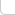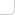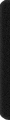# 某岛

… : "…アッカリ～ン . .. . " .. .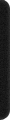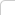March 29, 2011

# UVa 756. Biorhythms

### Breif description :

Give you a system of modular arithmetic equation ..

### Analyse :

Solve the system of the modular arithmetic equation is a fundament in Number Theroy, There is a method called Chinese Remainder Theorem could give us a good answer.

```#include
#include
using namespace std;

const int M1 = 23 , M2 = 28, M3 = 33, M = M1 * M2 * M3;
int m1, m2, m3, mm1, mm2, mm3, c1, c2, c3;
int r1, r2, r3, x0;
int n, a;

inline void _M(int &a){
if (a < 0) a += M;
a %= M;
}

inline int _M(const int& a, const int& n){
if (a < 0) return a + n;
return a;
}

inline int _I(int b, const int& n){
int a = n;
int x1 = 0, x2 = 1, q;
while (true){
q = a / b, a %= b;
if (!a) return _M(x2, n);
x1 -= q * x2;
q = b / a, b %= a;
if (!b) return _M(x1, n);
x2 -= q * x1;
}
}

inline int _CRT(){
int res = r1 * c1; _M(res);
res += r2 * c2, _M(res);
res += r3 * c3, _M(res);
res -= x0, _M(res);
return res;
}

int main(){
m1 = M / M1, m2 = M / M2, m3 = M / M3;
mm1 = _I(m1, M1), mm2 = _I(m2, M2), mm3 =  _I(m3, M3);
c1 = _M(m1 * mm1, M), c2 = _M(m2 * mm2, M), c3 = _M(m3 * mm3, M);

int T = 0;
while (cin >> n && n!=0){
printf("Case %d: the next triple peak occurs in %d days.\n", ++T, _CRT() ? _CRT() : M);
}
}
```

### Further discussion :

This problem has fixed modulus, which makes it more appropriate to do so, but … Implement the algorithm naively according to the original CRT has a few restriction.[nbnote ]

Here have another method called Merge-Equation Could extend its scope, which is equivalence on principle, different on motivation …
[/nbnote]

Here we give another iteration algorithm :

``` int x = r, step = m;
for (int i = 1; i < n; i ++){
while ((x - a[i]) % m[i] != r[i])
x += step;
step = lcm(step, m[i]);
}```

This algorithm is base on the brute force enumerate method, The inner while loop actually is to solve the following equation:

x + ? step = r[i] (mod m[i])

... And this step could work out with a exEuclid algorithm which is similar to the previous one...

The method maybe more straight and powerful (faster?), to taste those subtle distinction, try UVa 700. Date Bugs ... (or Poj ???)